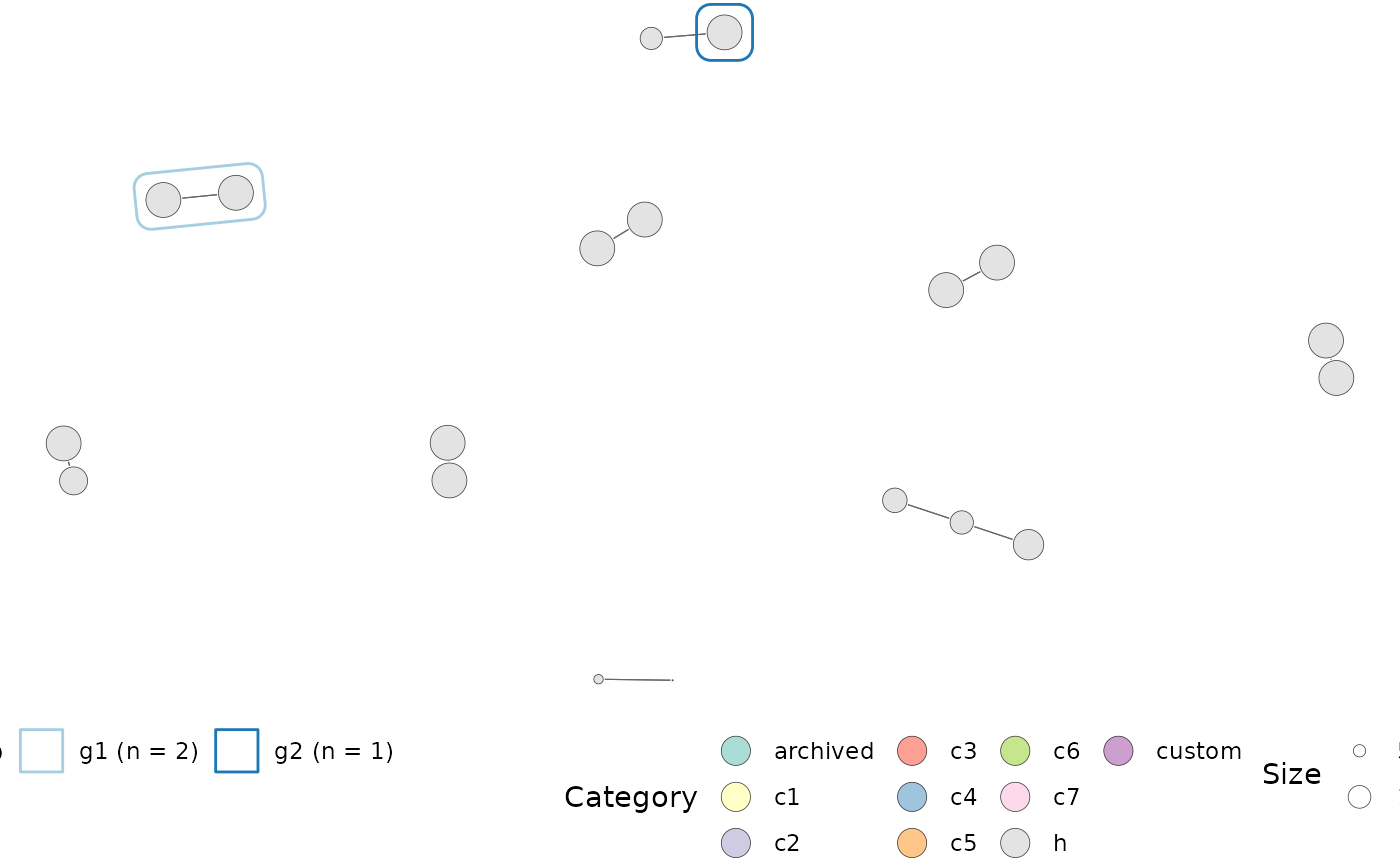Plots a network of gene set overlap with overlap computed using the computeMsigOverlap() and a graph created using computeMsigNetwork().

plotMsigNetwork(
ig,
markGroups = NULL,
genesetStat = NULL,
nodeSF = 1,
edgeSF = 1,
lytFunc = "graphopt",
lytParams = list()
)

Arguments

ig

an igraph object, containing a network of gene set overlaps computed using computeMsigNetwork().

markGroups

a named list, of character vectors. Each element of the list represent a group and contains a character vector with node names. Up to 12 groups can be visualised in the plot.

genesetStat

a named numeric, statistic to project onto the nodes. These could be p-values, log fold-changes or gene set score from a singscore-based analysis.

nodeSF

a numeric, indicating the scaling factor to apply to node sizes.

edgeSF

a numeric, indicating the scaling factor to apply to edge widths.

lytFunc

a character, specifying the layout to use (see ggraph::create_layout()).

lytParams

a named list, containing additional parameters needed for the layout (see ggraph::create_layout()).

a ggplot2 object

Examples

data(hgsc)
ovlap <- computeMsigOverlap(hgsc, thresh = 0.15)
ig <- computeMsigNetwork(ovlap, hgsc)
groups <- list(
'g1' = c("HALLMARK_HYPOXIA", "HALLMARK_GLYCOLYSIS"),
'g2' = c("HALLMARK_INTERFERON_GAMMA_RESPONSE")
)

plotMsigNetwork(ig, markGroups = groups)